# Y4 Maths Task- Tuesday 24th March

Fractions and decimals

You might need to work out on paper and then write it in your reply. If you have a printer, you could print it out and write your answers on there first.

This activity should take around an hour.

Click on the blue link to see the work more clearly.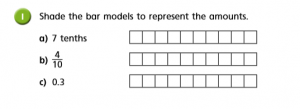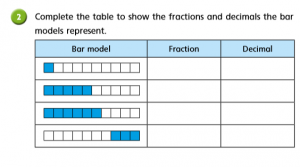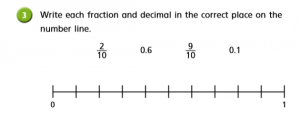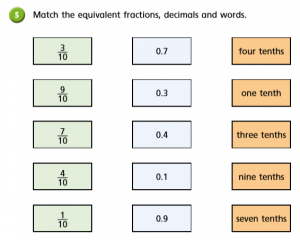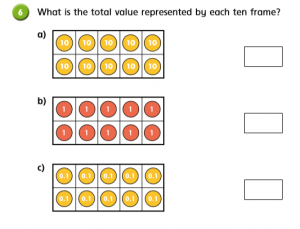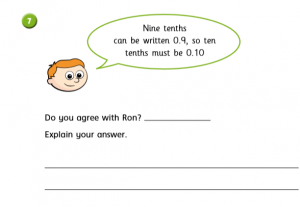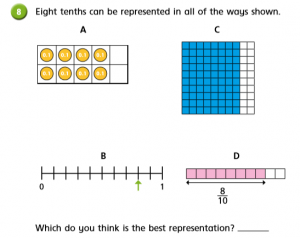maths lesson 2

### 17 thoughts on “Y4 Maths Task- Tuesday 24th March”

•24th March 2020 at 11:36 am

1.
A) 7 sqares
B) 4 sqares
C) 3 sqares
2.
a) 1/10 or 0.1
b) 5/10 or 0.5
c) 6/10 or 0.6
d) 3/10 or 0.3
3.
a) 0.1
b) 2/10
c) 0.6
d) 9/10
4.
a) 0.3 or 3/10
b) 0.4 or 4/10
c) 0.7 or 7/10
5.
3/10 or 0.3 or three tenths
9/10 or 0.9 or nine tenths
7/10 or 0.7 or seven tenths
4/10 or 0.4 or four tenths
1/10 or 0.1 or one tenths
6.
A) 10/10
b) 10/10
c) 10/10
7.
This is wrong because it can also be writon as 0.1
8. I think the one with the shaded squares because you can count and there will be 8/10

•24th March 2020 at 11:40 am

Great reasoning Brooke. Time for a break

•24th March 2020 at 11:37 am

1.
a] 7 squares
b] 4 squares
c] 3 squares

2.
a] 1/10= 0.1= 0.10
b] 5/10=1/2= 0.5
c] 6/10 =0.6
d] 3/10 =0.3

3.
a] 0.1,2/10,0.6,9/10

4.
a] 0.3 or 3/10
b] 0.1 or 1/10
c] 0.3 or 3/10

5.
a] four tenths = 0.4
b] one tenths = 0.1
c] three tenths = 0.3
b] nine tenths = 0.9
d] seven tenths =0.7

6.
a] 10/10
b] 10/10
c]10/10

7.
a] ron is wrong because you can’t do 0.10

8. it is because you can count to it then just say 0.8

•24th March 2020 at 11:40 am

great understanding of fractions. Question 8 was which way of showing tenths do you prefer and why?

•24th March 2020 at 11:51 am

2: Bar model 1: Fraction: 1/10. Decimal: 0.1. Bar model 2: Fraction: 5/10. Decimal: 0.5. Bar model 3: Fraction:6/10. Decimal: 0.6. Bar model 4: Fraction: 3/10. Decimal: 0.3.
3: 0.1 is 1 along from 0. 2/10 is 2 along from 0. 0.6 is 6 along from 0. 9/10 is 9 along from 0.
4: A: 3/10 or 0.3. B: 4/10 or 0.4. C: 7/10 or 0.7.
5: 3/10,0.3, three tenths. 9/10, 0.9, nine tenths. 7/10, 0.7, seven tenths. 4/10,0.4, four tenths. 1/10, 0.1, one tenth.
6: a:100. b:10. c:1.
7 I disagree with Ron because ten tenths would be a whole, so it wouldn’t be 0.10, it would be 1.0.
8: I think D is the best.

•24th March 2020 at 1:27 pm

Great understanding Ben, great to see you on here. Hope you are enjoying the sunshine.

•24th March 2020 at 11:56 am

1.
a. 7 tenths = 7 squares b.
1 1 1 1 1 = 10/1
1 1 1 1 1
b. 4/10 = 4 squares
c. 0.3 = 3 squares c.
0.1 0.1 0.1 0.1 0.1
0.1 0.1 0.1 0.1 0.1
2.
Fraction
1/10 0.1
5/10 0.5
6/10 0.6
3/10 0.3
3.
0.1
2/10
0.6
9/10
4.
A 0.3 or 3/10
B 0.4 or 4/10
C 0.7 or 7/10
5.
3/10 0.3 three tenths
9/10 0.9 nine tenths
7/10 0.7 seven tenths
4/10 0.4 four tenths
1/10 0.1 one tenth
6.
A. 10 10 10 10 10 = 10/10
10 10 10 10 10

7. No i dont agree with Ron because it would look like 10/10.
8. I would choose C because it is shown in a clear way for people and it is obvious it is 8 squares.

•24th March 2020 at 11:58 am

1. A 7 Squares =4 squares
b. 0.3 = 3 squares
c. 4/10
2. 1/10 = 0.10
3 b 2/10 9/10 0.6
4. a 0.3 or 3 tenths
b . 0.4 or 4 tenths
c. 0.7 or 7 tenths
5. 3/10 = 0.3 =3 tenths
9/10 = 0.9 =nine tenths
7/10 = 0.7 = seven tenths
4/10 = 4.0 = 4 tenths
1/10 = 0.1 = one tenth

•24th March 2020 at 12:01 pm

Good understanding of decimals and fractions

•24th March 2020 at 1:54 pm

1: a = 7 squares
b= 4 squares
c= 3 squares

2: a = 1/10 or 0.1
b = 5/10 or 0.5
c = 6/10 or 0.6
d = 3/10 or 0.3

3: 0.1, 2/10,, 6/10, 0.9

4: a = 3/10 or 0.3
b = 4/10 or 0.4
c = 7/10 or 0.7

5: 3/10 or 0.3 or three tenths
9/10 or 0.9 or nine tenths
7/10 or 0.7 or seven tenths
4/10 or 0.4 or four tenths
1/10 or 0.1 or one tenth

6: a = 100
b = 10
c = 1

7: this is wrong as he done 0.1 not 1

8: I think it’s the shaded one as it’s easier to understand

•24th March 2020 at 2:16 pm

Great understanding Owen

•24th March 2020 at 2:09 pm

1.
a) 7 tenth =7 squares
b)4 tenths=4 squares
y
c)0.3=3 squares shaded at the back

2.
fraction decimal-yes
yes
yes
yes

3.
0.1-2 tenths-0.6-9 tenths

4.
A=4 tenths or 0.4
B=5 tenths or 0.5
C=8 tenths or 0.8

5.
3-10 goes to 0.3 goes to three tenths
9-10 goes to 0.9 goes to nine tenths
7-10 goes to 0.7 goes to seven tenths
4-10 goes to 0.4 goes to four tenths
1-10 goes to 0.1 goes to one tenths

6.
100
10
0.10

7.
yes because 1-10 and 1-9 can be 0.10 or 0.9 and there decimals

8.
the best is c
6 squares
0.1+0.1+0.1+0.1+0.1+0.1

•24th March 2020 at 2:15 pm

Great understanding of decimals and Fractions Kristers

•26th March 2020 at 4:53 pm

2a) 1/10, 0.1
b) 5/10, 0.5
c) 3/10, 0.3
3) 0.1, 2/10, 0.6, 9/10
4 a) 3/10 or 0.3
b) 4/10 or 0.4
c) 7/10 or 0.7
5) 3/10- 0.3- three tenths
9/10- 0.9- 9 tenths
7/10- 0.7- seven tenths
4/10- 0.4- four tenths
1/10- 0.1- one tenths
6) 1.0
0.1
0.01
7) He is incorrect. 10tenths as a fraction is 10/10 so in decimal it will be 1.00.
8) C

•28th March 2020 at 6:50 pm

1.
a) 7 squares
b) 4 squares
c) 3 squares
2.
a) 1/10 = 0.1
b) 5/10 = 0.5
c) 6/10 = 0.6
d) 3/10 = 0.3
3.
a) 0.1
b) 2/10
c) 0.6
d) 9/10
4.
a) 3/10 or 0.3
b) 4/10 or 0.4
c) 7/10 or 0.7
5.
3/10 or 0.3 or three tenths
9/10 or 0.9 or nine tenths
7/10 or 0.7 or seven tenths
4/10 or 0.4 or four tenths
1/10 or 0.1 or one tenths
6.
a) 100
b) 10
c) 1
7.
I don’t agree with Ron as 10/10 is 1, not 0.10
8. a b c d
The best representation is D

•29th March 2020 at 3:09 pm

Brilliant. I like that you have remembered to include the fraction and decimal equivalent.

•23rd April 2020 at 3:46 pm

1.
A) 7 sqares
B) 4 squares
C) 3 squares
2.
a) fraction 1/10 decimal 0.1
b) fraction 5/10 decimal 0.5
c) fraction 6/10 decimal 0.6
d) fraction 3/10 decimal 0.3
3.
a) 0.1 b) 2/10 c) 0.6 d) 9/10
4.
a) 0.3 or 3/10
b) 0.4 or 4/10
c) 0.7 or 7/10
5.
3/10 or 0.3 three tenths
9/10 or 0.9 nine tenths
7/10 or 0.7 seven tenths
4/10 or 0.4 four tenths
1/10 or 0.1 one tenths
6.
A) 100
b) 10
c) 1
7.
No, because 10 tens equals to one
8. I prefer squares as you can see and squares